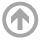Naval Architecture & Ocean Engineering# EN330: Probability and Statistics with Ocean Applications

## Catalog Description

### EN330: Probability and Statistics with Ocean Applications (3-0-3)

The course is designed to give a basic understanding of probability and statistics, as related to engineering problem solving, with an emphasis on marine applications.  Topics covered include basic descriptive statistics, probability analysis, probability distributions, uncertainty estimation, time-series analysis, confidence limits, and regression analysis. While the course involves a blend of theory and analytics, practical applications of probability and statistics to engineering in the marine environment will be emphasized.  Additionally, this course covers the general topics of the Fundamentals of Engineering (FE) exam.

### Textbook

• Basic Statistics (Lind, Marchal, and Wathen)

### Goals

1. Understand and relate fundamental concepts of statistics and probability to real-world engineering applications, particularly those pertaining to the marine environment.
2. Develop and implement computer algorithms to analyze and display random data (in the form of histograms, cumulative distributions, sea-surface elevations, etc.) for purposes of engineering decision-making.
3. Assess uncertainties in data sampling through statistical inference techniques to develop point estimates and confidence intervals for estimation of the (population’s) mean and variance.
4. Apply knowledge of probability and statistics to develop a statistical description of the random sea surface, including application of the Gaussian distribution to water surface elevation and the Rayleigh distribution to wave heights.
5. Relate to all topics covered on the Fundamentals of Engineering (FE) exam – Probability and Statistics portion – including a familiarity with the FE reference manual and an ability to answer FE-approved sample problems in a time-efficient manner.

### Prerequisites

• SM212, Differential Equations, AND
• EN245, Principles of Ocean Engineering Systems, OR
• EN247, Principles of Naval Architecture and Marine Engineering

### Class Topics

1. Histograms and Frequency Diagrams
2. Statistics for Central Tendency and Dispersion
3. Basic Probability – Sample Spaces, Sets, Events
4. Addition & Multiplication Probability Rules
5. Conditional Probability and Probability Trees
6. Theorem of Total Probability & Bayes Theorem
7. Discrete Probability Distributions – Binomial, Poisson
8. Continuous Probability Distributions – General PDFs & CDFs
9. Continuous Probability Distributions – Uniform, Exponential, Normal, Rayleigh
10. Linear Combinations of Multiple Variables
11. Analysis of Sea Surface Elevation
12. Zero-Crossing Analysis of Wave Heights
13. Extreme Value Analysis of Wave Heights
14. Sampling & Central Limit Theorem
15. Confidence Limits – Student t and Chi-Squared
16. Hypothesis Testing
17. Regression – Method of Least Squares
18. Correlation and Goodness of Fit

### Validation

Midshipmen studying abroad may be eligible to earn credit for this course.  This must be approved by the Course Coordinator and the Midshipman's Academic Adviser.  To be considered for validation:

1. Review the Tutorial
1. Random Wave Analysis (Supplemental Paper)
2. Statistics & Probabilities of the Water Surface (Supplemental Paper)
3. Rayleigh Probability Distribution Applied to Random Wave Heights (Supplemental Paper)
2. Complete Problem Set #1
3. Complete Problem Set #2